• 稀疏矩阵压缩存储
2021-05-29 15:36:01
1. 编写程序任意输入一个稀疏矩阵，用三元组顺序表压缩存储稀疏矩阵。
2. 对稀疏矩阵进行转置，输出转置后的矩阵。

代码实现：

#include<stdlib.h>
#include<iostream>
#include<stdio.h>
using namespace std;
#define Maxsize 12500
using namespace std;
typedef int status;
typedef int ElemTpye;
typedef struct {
int i, j;
ElemTpye e;
}Triple;
typedef struct {
Triple data[Maxsize];
int mu, nu, tu;
}TsMatrix;
status GreatTriple(TsMatrix& T)
{
int m, n, t;
cout << "请输入行数:";
cin >> m;
cout << "请输入列数：";
cin >> n;
cout << "请输入非0元个数：";
cin >> t;
int i, j, e, p = 0;
cout << "请输入矩阵三元表。" << endl;
while (p < t)
{

scanf_s("%d%d%d", &i, &j, &e);
if (e != 0)
{
T.data[p].i = i;
T.data[p].j = j;
T.data[p].e = e;
p++;
}
}
T.mu = m; T.nu = n; T.tu = t;
return 0;
}
status Transpose(TsMatrix T, TsMatrix& t)
{
t.mu = T.nu; t.nu = T.mu; t.tu = T.tu;
if (t.tu)
{
int k = 0;
for (int i = 0; i < t.mu; i++)
{
for (int j = 0; j < t.nu; j++)
{
if (T.data[j].j == i)
{
t.data[k].i = T.data[j].j;
t.data[k].j = T.data[j].i;
t.data[k].e = T.data[j].e;
k++;
}
}
}
}
return 0;
}

status Show(TsMatrix T)
{
if (T.tu <= 0)
{
cout << "这是一个空矩阵" << endl;
return -1;
}
cout << "矩阵为：" << endl;
int p = 0;
for (int i = 0; i < T.mu; i++)
{
for (int j = 0; j < T.nu; j++)
{
if (T.data[p].i == i && T.data[p].j == j)
{
cout << T.data[p].e << "    ";
++p;
}
else cout << "0" << "    ";
}
cout << endl;
}
return 1;
}
int main()
{
cout << "创建矩阵:" << endl;
TsMatrix T, t;
GreatTriple(T);
cout << endl;
cout << "打印矩阵:" << endl;
Show(T);
Transpose(T, t); cout << "转置后"; Show(t);
return 0;
}


运行结果：数据结构 c++ 算法 链表
更多相关内容
•C语言
• 本篇文章以五子棋的棋局保存为背景，用c语言实现原始稀疏矩阵转换为压缩矩阵并且存储为文件，棋局复盘则读取文件将压缩矩阵转换为原始稀疏矩阵。 图解 假如有如下棋局，如果要保存，可以用1来表示红棋子，2来表示...

## 背景需求

在一个矩阵中，如果非0元素远远少于0元素，那么这个矩阵就是稀疏矩阵，在实际应用中，计算机会耗费大量的空间存储这些无意义的0元素，如果能够压缩一下，将会减少计算机的开销。本篇文章以五子棋的棋局保存为背景，用c语言实现原始稀疏矩阵转换为压缩矩阵并且存储为文件，棋局复盘则读取文件将压缩矩阵转换为原始稀疏矩阵。

## 图解

假如有如下棋局，如果要保存，可以用1来表示红棋子，2来表示黑棋子，0表示无棋子。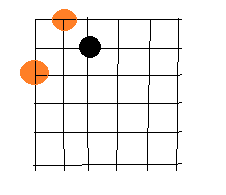需求就是将上述棋局转换为如下矩阵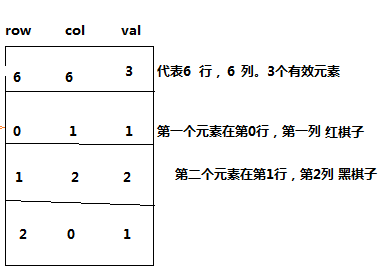## 算法

棋局保存：1、统计有多少个有效元素count

2、定义长度为count+1，宽度为3的矩阵arr

3、逐一赋值，存入文件

棋局复盘：1、读取文件第一行，得到原始矩阵的行列数，定义原始矩阵

2、将文件中对应的非0值赋值给原始矩阵

## 棋局保存代码实现

#include <stdio.h>
#include <stdlib.h>
#define LEN 6
/**
求输出稀疏矩阵
*/
void showArr(int *arr, int row,int col){
int num = row*col;
int cnt = 0;
for(int i = 0 ; i < num ; i++){

printf("%4d",*(arr+i));
cnt++;
if(cnt%3==0) printf("\n");
}
}
/**
求输出原始方阵
*/
void showoldArr(int arr[][LEN]){
for(int i = 0 ; i < LEN ; i++){
for(int j = 0 ; j < LEN ; j++){
printf("%4d",arr[i][j]);
}
if(j == LEN) printf("\n");
}
}
/**
求有效元素个数
*/
int arrNum(int arr[][LEN]){
int count = 0;
for(int i = 0 ; i < LEN ; i++){
for(int j = 0 ; j < LEN ; j++){
if(arr[i][j]!=0) count++;
}
}
return count;
}
int main(){
int i = 0,j=0,k = 0,m = 0;
int oldArr[LEN][LEN] = {{0,1},{0,0,2},{1}};
//1表示白棋子，2表示黑棋子，0表示无棋子
printf("原始矩阵：\n");
showoldArr(oldArr);
//得到有效数据的个数，确定稀疏矩阵的行数
int num = arrNum(oldArr);
int row = num+1;
int col = 3;
//创建稀疏矩阵,因为c语言中的二维数组长度不能含有变量，所以用指针的指针来定义
int  *newArr;
newArr=(int *)malloc(sizeof(int)*(num+1)*3);

//先给稀疏矩阵的第一行赋值
*newArr = LEN;
*(newArr+1) = LEN;
*(newArr+2) = num;
//再给剩下的行赋值
for(i = 3 ; i < row*col ; i+=3){
for(; k < LEN ; ){
for(m = 0 ; m < LEN ; m++){
if(oldArr[k][m]!=0){
*(newArr+i) = k;
*(newArr+i+1) = m;
*(newArr+i+2) = oldArr[k][m];
}
}
k+=1;
break;
}
}
printf("稀疏矩阵：\n");
showArr(newArr,row,col);
//将矩阵写入文件
FILE *p=fopen("arr.txt","w");
for(i = 0 ; i < row*col ;){

fprintf(p,"%d",*(newArr+i));
i++;
if(i%3==0&&i!=row*col) fprintf(p,"%s","\n");
}

fclose(p);

}

棋局保存运行结果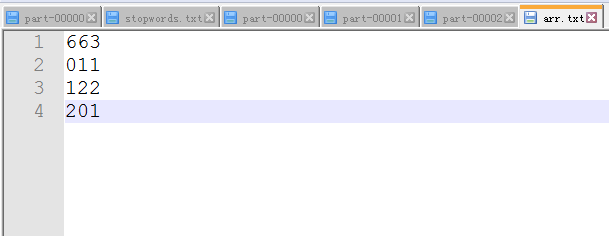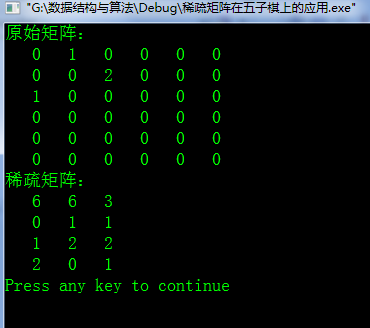## 棋局复盘代码实现

#include <stdio.h>
#include <stdlib.h>
/**
输出原始数组
*/
void showArr(int *arr, int row,int col){
int num = row*col;
int cnt = 0;
for(int i = 0 ; i < num ; i++){

printf("%4d",*(arr+i));
cnt++;
if(cnt%row==0) printf("\n");
}
}
int main(){
char line;

FILE *f = fopen("arr.txt", "rb");
//先读第一行
fgets(line, 256, f);
int ele =	atoi(line);
char rlen =ele/100;			//得到矩阵长度
char clen =ele/10%10;		//得到矩阵宽度
int *arr;	//定义一个空间来放原始矩阵
arr = (int *)malloc(sizeof(int)*rlen*clen);//根据矩阵长度宽度开辟空间
for(int j = 0;j<rlen*clen;j++){*(arr+j) =0;} //先将所有的值赋值为0

while (!feof(f) && !ferror(f)) {	//开始按行读取数据
fgets(line,sizeof(line),f);

for(int i = 0;i<rlen*clen;i++){

int ele =atoi(line);
int row = ele/100;	//得到行
int col = ele/10%10;	//得到列
int val = ele%10;//得到值

if((row*rlen+col)==i)	//如果此时的行列正好是目标行列，赋值即可
*(arr+i) = val;
}
}
showArr(arr,rlen,clen);
fclose(f);
return 0;
}



## 棋局复盘运行结果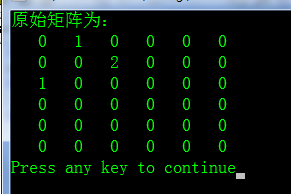## 总结

c语言中，数组的长度定义不可以为变量，必须是常量，因此这里便用指针开辟空间来代替二维数组，实际上二维数组的物理内存和一位数组一样，都是线性存储，因此实质上是一样的。指针的取值怎么取也是容易出错的地方，如果写错表达式，会出现程序停止。

展开全文c语言
• 压缩存储稀疏矩阵的时候我们只存储极少数的有效数据。我们在这里使用三元组存储每一个有效数据，三元组按原矩阵中的位置，以行优先级先后次序依次存放。下面我们来看一下代码实现。 #include #include #include ...
• 主要介绍了C++ 实现稀疏矩阵压缩存储的实例的相关资料,M*N的矩阵，矩阵中有效值的个数远小于无效值的个数，且这些数据的分布没有规律，需要的朋友可以参考下
• 什么是稀疏矩阵：在矩阵中，我们常见的都是稠密矩阵，即非0元素数目占大多数时；若数值为0的元素数目远远多于非0元素的数目，并且非0元素分布没有规律时，则称该矩阵为稀疏矩阵。与之相区别的是，如果非零元素的分布...什么是稀疏矩阵：

在矩阵中，我们常见的都是稠密矩阵，即非0元素数目占大多数时；若数值为0的元素数目远远多于非0元素的数目，并且非0元素分布没有规律时，则称该矩阵为稀疏矩阵。与之相区别的是，如果非零元素的分布存在规律(如上三角矩阵、下三角矩阵、对角矩阵)，则称该矩阵为特殊矩阵。

下图1为一个稀疏矩阵的示例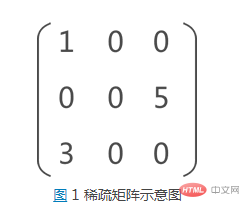稀疏因子是用于描述稀疏矩阵的非零元素的比例情况。设一个n*m的稀疏矩阵A中有t个非零元素，则稀疏因子δ的计算公式如下：δ=t/(n∗m), 当这个值小于等于0.05时，可以认为是稀疏矩阵

稀疏矩阵的存储方式

存储矩阵的一般方法是采用二维数组，其优点是可以随机地访问每一个元素，因而能够较容易地实现矩阵的各种运算。但对于稀疏矩阵而言，若用二维数组来表示，会重复存储了很多个0了，浪费空间，而且要花费时间来进行零元素的无效计算。所以必须考虑对稀疏矩阵进行压缩存储。

稀疏矩阵的压缩存储，数据结构提供有 3 种具体实现方式：三元组顺序表；

行逻辑链接的顺序表：可以看作是三元组顺序表的升级版，即在三元组顺序表的基础上改善了提取数据的效率。

十字链表；

想要了解更多相关知识，请关注 html中文网！！

展开全文• c 语言实现 稀疏矩阵 的创建 和 C语言 压缩存储 压缩矩阵转置
• eg:规定无效数据为 01 0 0 0 00 0 0 0 20 3 0 0 04 0 0 0 0上述矩阵则可称为一个稀疏矩阵我们在学习C语言的时候已经见过并使用过矩阵，其实它在我们的编程语言里可以翻译成二维数组，由于稀疏矩阵的有效数据十分的少...

矩阵(Matrix)是一个按照长方阵列排列的复数或实数集合.

稀疏矩阵：有效数据远少于无效数据。

eg:规定无效数据为 0

1 0 0 0 0

0 0 0 0 2

0 3 0 0 0

4 0 0 0 0

上述矩阵则可称为一个稀疏矩阵

我们在学习C语言的时候已经见过并使用过矩阵，其实它在我们的编程语言里可以翻译成二维数组，由于稀疏矩阵的有效数据十分的少，完全存储十分耗费我们的空间，所以我们选择只存储它的有效数据位，所以我们可以直接使用一维数组将其存储起来，但是我们必须让别人在看它时还能知道它是一个矩阵，于是我们必须将其所在的位置使用一个三元组存储起来！

三元组的定义如下：template

struct Triple

{

T _value;//有效值

int _row;//该值所在行

int _col;//该值所在列

Triple(const T& data = T(), int m = 0, int n = 0)

:_value(data),_row(m), _col(n)

{}

};

矩阵的定义如下：class SpaMatrix

{

public:

SpaMatrix(T* arr = NULL, int m = 0, int n = 0, int inva = 0)

:_Row(m), _Col(n), _invalid(inva)

{

int index = 0;

for (int i = 0; i

{

for (int j = 0; j

{

if (arr[i*n + j] != _invalid)

{

_a.push_back(Triple(arr[i*n + j], i, j));

_n ++;

}

}

}

}

private:

vector > _a;//存储三元组

int _invalid;//规定的无效值

int _Row;//矩阵的行数

int _Col;//矩阵的列数

};

在矩阵里，我们经常能够看见一个运算，就是矩阵的转置，即使得a[i][j] = a[j][i]，我们之前在存储矩阵时选择了行优先的存储方式，但是，转置之后，我们的矩阵是以原来的行为它的列，我们该如何做呢？

方法一：普通转置

**我们遍历矩阵，将第一列数据先放入新的矩阵，再放第二列，第三列，以此类推，这样，我们就能够得到新的矩阵

下面是代码的实例(截取重要部分代码)：//普通转置

SpaMatrix::SpaMatrix OrdinaryTrans()

{

int count = 0;

int index = 0;

SpaMatrix sp;//使用一个临时变量存储转置后的矩阵

for (int j = 0; j

{

for (int index = 0; index

{

if (_a[index]._col == j)

{

//每次只push需要的列

sp._a.push_back(_a[index]);

sp._a[index]._row = _a[index]._col;

sp._a[index]._col = _a[index]._row;

}

}

}

//更新转置后的矩阵行列信息

sp._Row = _Col;

sp._Col = _Row;

return sp;

}

方法二：快速转置

**我们将原来的矩阵的每一列有效数据的起始位和每一列有效数据的个数保存起来，作为新矩阵的每一行有效数据的起始位置和有效数据个数，当我们想要的得到某个数据时，使用 上一行的起始位置+有效数据的个数即可得到我们新的矩阵。

我们来看看代码的实例：//快速转置

SpaMatrix::SpaMatrix FastTrans()

{

//统计有效数据的开始位置

int *RowStart = new int[_Col];

//统计转置之后的矩阵里每行的有效数据的个数

int *RowCount = new int[_Col];

memset(RowCount, 0, sizeof(int)*_Col);

memset(RowStart, 0, sizeof(int)*_Col);

size_t index = 0;

//Set RowCount

while (index

{

RowCount[_a[index]._col]++;

index++;

}

//Set RowStart

RowStart = 0;

for (int i = 1; i

{

RowStart[i] = RowStart[i - 1] + RowCount[i - 1];

}

//构造转置之后的矩阵

SpaMatrix sptrans;

sptrans._Row = _Col;

sptrans._Col = _Row;

sptrans._n = _n;

//index 值已经改变了，必须重新让其等于0

index = 0;

//此处使用下标访问必须开辟空间，但如果使用push_back()可以不用开辟~

sptrans._a.resize(_a.size());

while(index

{

int Rowindex = _a[index]._col;

//此处注意引用

int& RowSt = RowStart[Rowindex];

sptrans._a[RowSt]._value = _a[index]._value;

sptrans._a[RowSt]._col = _a[index]._row;

sptrans._a[RowSt]._row = _a[index]._col;

index++;

//每次必须更新该位置，否则错误

RowSt++;

}

return sptrans;

}

以上是我自己实现的转置代码，虽然代码渣，但是就是有迷之自信敢给你们看，我就问你们怕不怕展开全文• 实现稀疏矩阵压缩存储，并实现矩阵转置和求和。 输入矩阵时，首先需要输入非零元素的个数，然后分别输入矩阵的 行号，列号和值。 输完2个矩阵后，自动进行计算第一个矩阵的转置以及两个矩阵的和。 例如：输入如下...c++
• value=[1,2]value=[1,2]value=[1,2]，表示元素值 col=[1,2]col=[1,2]col=[1,2]，表示元素值列标 row=[1,2]row=[1,2]row=[1,2]，表示元素值行标 #2、CSR(Compressed Sparse Row Format 压缩稀疏行格式) 例如： A=...数据结构
•java
• #include #define OK 1 #define MAX 10 typedef struct { int i,j; int v; }TriTupleNode; typedef struct { TriTupleNode data... printf("稀疏矩阵转置后:\n"); TransposeMatrix(a,b); ShowMatrix(pb); return 0; }
• 数据结构中，提供针对某些特殊矩阵的... 含有大量 0 元素的矩阵，比如稀疏矩阵、上（下）三角矩阵； 针对以上两类矩阵，数据结构的压缩存储思想是：矩阵中的相同数据元素（包括元素 0）只存储一个。 对称矩阵 ...
• 一、稀疏矩阵的定义　矩阵中非零元素的个数远远小于矩阵元素的总数，并且非零元素的分布没有规律，通常认为矩阵中非零元素的总数比上矩阵所有元素总数的值小于等于0.05时，则称该矩阵为稀疏矩阵(sparse matrix)，该...机器学习
• ## 稀疏矩阵的压缩存储方法

万次阅读 多人点赞 2019-06-02 22:26:51
1 什么是稀疏矩阵： 在矩阵中，我们常见的都是稠密矩阵，即非0元素数目占大多数时；若数值为0的元素数目远远多于非0元素的数目，并且非0元素分布没有规律时，则称该矩阵为稀疏矩阵。与之相区别的是，如果非零元素的...
• 实际应用中会有很多利用高阶矩阵的问题，有的高阶矩阵已达到几十万阶，几千亿个元素。然而，多数的高阶矩阵中...否则称为随机稀疏矩阵。 未必所有的矩阵都可以压缩，要看其是否具备以上压缩条件 特殊形状矩阵的存储算法
• 1. 什么是稀疏矩阵？...稀疏矩阵压缩存储的原则？ 只存储矩阵的行列维数，以及每个非零元的行列下标及其值。 例如，稀疏矩阵M如下： 3.三元组顺序表 （1）三元组顺序表类型描述 typedef int ElementType; type...
• 5.3稀疏矩阵压缩存储上 稀疏矩阵是指矩阵中大多数元素为零的矩阵。 从直观上讲，当非零元素个数低于总元素的 30 %时,这样的矩阵为稀疏矩阵。如下图所示的矩阵 M、 N 中,非零元素个数均为 8 个, 矩阵元素总数均为 6 ...数据结构
• 对称矩阵及稀疏矩阵压缩存储 1.稀疏矩阵 　对于那些零元素数目远远多于非零元素数目，并且非零元素的分布没有规律的矩阵称为稀疏矩阵（sparse）。 　人们无法给出稀疏矩阵的确切定义，一般都只是凭个人的直觉来...
• 稀疏矩阵压缩存储思想： -存储非零元：值；位置（行列号） -存储适当的辅助信息：行数；列数；非零元的个数 三元组&lt;i,j,e&gt; 0 12 9 0 0 0 0 0 0 0 0 0 0 0 -3 0 0 0 0 14 0 0 0 ...十字链表
• 稀疏矩阵压缩存储实现-三元组1 数据结构设计与头文件编写1.1 数据结构设计1.2 数据结构实现1.3 核心代码编写2 代码测试2.1 数据结构基本操作测试2.2 核心代码测试*3 头文件完整定义 1 数据结构设计与头文件编写 &...数据结构 算法 知识图谱 三元组
• 【定义】 所谓稀疏矩阵，假设在m×n矩阵中，有t个元素不为零，令δ=t/(m×n),δ为矩阵的稀疏因子，如果δ≤0.05，则称矩阵为稀疏...在进行压缩存储的过程中，我们可以只存储稀疏矩阵的非零元素，为了表示非零元素...数据结构与算法 数组
•数据结构...